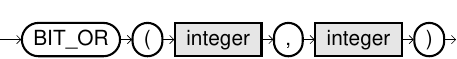# BIT_OR

## Purpose

This function computes the bitwise OR operation of two numerical values.

## Syntax

bit_or::=## Usage Notes

• Bit functions are limited to 64 bits, which is positive numbers between 0 and 18446744073709551615.
• The result data type is DECIMAL(20,0).
• If one of the parameters is NULL, the result is NULL.

## Example

``-- 1001 OR 0011 = 1011SELECT BIT_OR(9,3);``